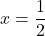Question

what choices are solutions for equation x^2-3x = -5/4

1.Here’s the solution,

=》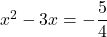=》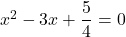now, let’s multiply the whole equation with 4

=》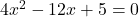=》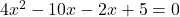=》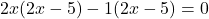=》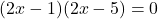so,

Case 1. where, 2x – 5 = 0

=》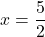case 2. where, 2x – 1 = 0

=》